# Chapter 8 8 2 Integration By Parts Let

• Slides: 35Chapter 8 8. 2 Integration By Parts Let G(x) be any antiderivative of g(x). In this case G’(x)=g(x), then by the product rule, Which implies Or, equivalently, as (1) The application of this formula is called integration by parts. Calculus, 8/E by Howard Anton, Irl Bivens, and Stephen Davis Copyright © 2005 by John Wiley & Sons, Inc. All rights reserved.In practice, we usually rewrite (1) by letting This yields the following alternative form for (1) Calculus, 8/E by Howard Anton, Irl Bivens, and Stephen Davis Copyright © 2005 by John Wiley & Sons, Inc. All rights reserved.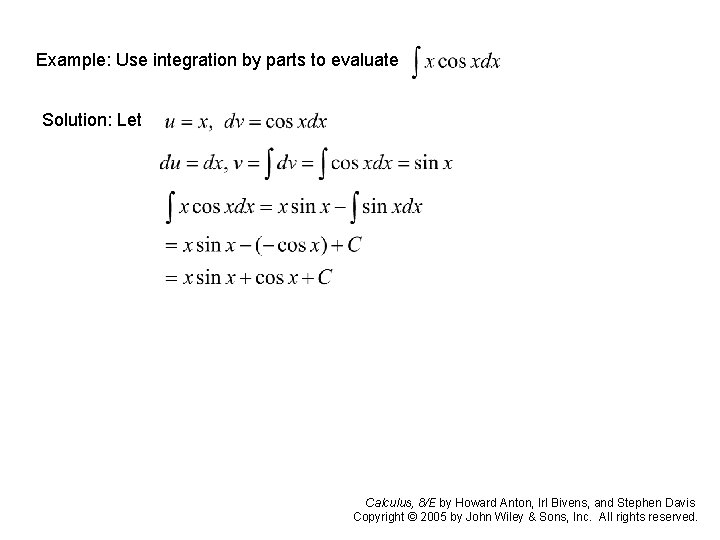Example: Use integration by parts to evaluate Solution: Let Calculus, 8/E by Howard Anton, Irl Bivens, and Stephen Davis Copyright © 2005 by John Wiley & Sons, Inc. All rights reserved.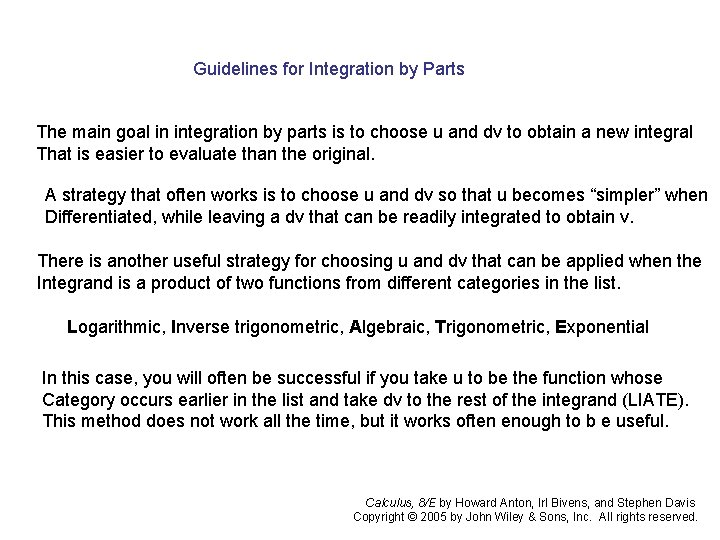Guidelines for Integration by Parts The main goal in integration by parts is to choose u and dv to obtain a new integral That is easier to evaluate than the original. A strategy that often works is to choose u and dv so that u becomes “simpler” when Differentiated, while leaving a dv that can be readily integrated to obtain v. There is another useful strategy for choosing u and dv that can be applied when the Integrand is a product of two functions from different categories in the list. Logarithmic, Inverse trigonometric, Algebraic, Trigonometric, Exponential In this case, you will often be successful if you take u to be the function whose Category occurs earlier in the list and take dv to the rest of the integrand (LIATE). This method does not work all the time, but it works often enough to b e useful. Calculus, 8/E by Howard Anton, Irl Bivens, and Stephen Davis Copyright © 2005 by John Wiley & Sons, Inc. All rights reserved.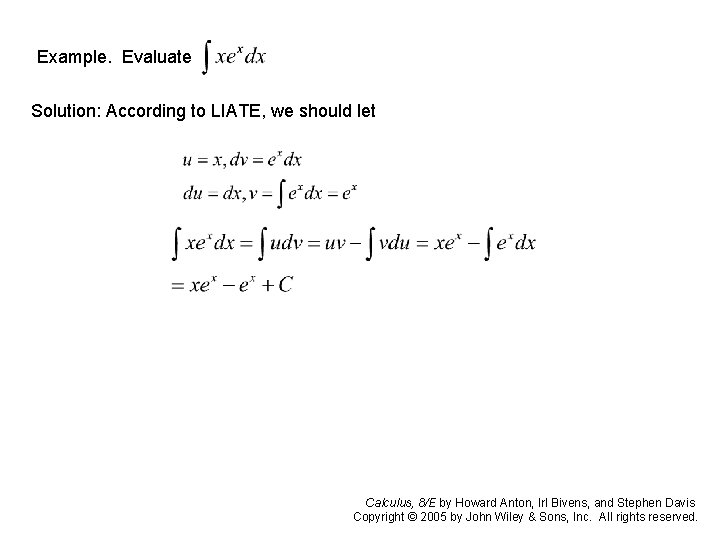Example. Evaluate Solution: According to LIATE, we should let Calculus, 8/E by Howard Anton, Irl Bivens, and Stephen Davis Copyright © 2005 by John Wiley & Sons, Inc. All rights reserved.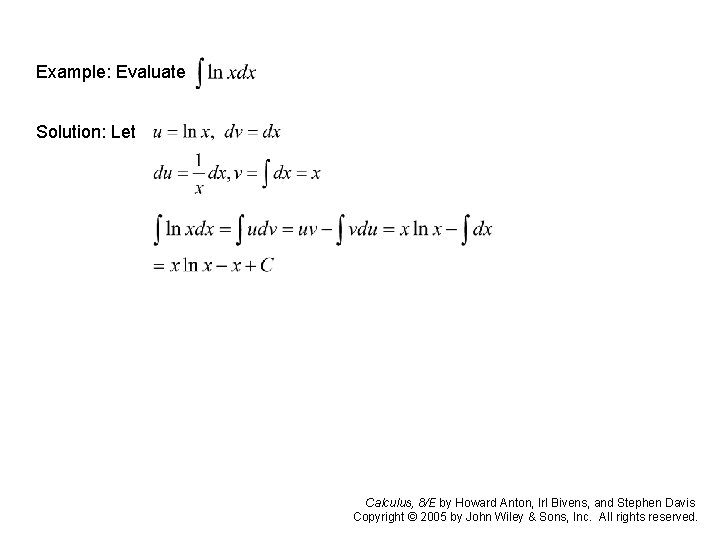Repeated Integration by Parts Example: Evaluate Solution: Let Apply integration by parts to Calculus, 8/E by Howard Anton, Irl Bivens, and Stephen Davis Copyright © 2005 by John Wiley & Sons, Inc. All rights reserved.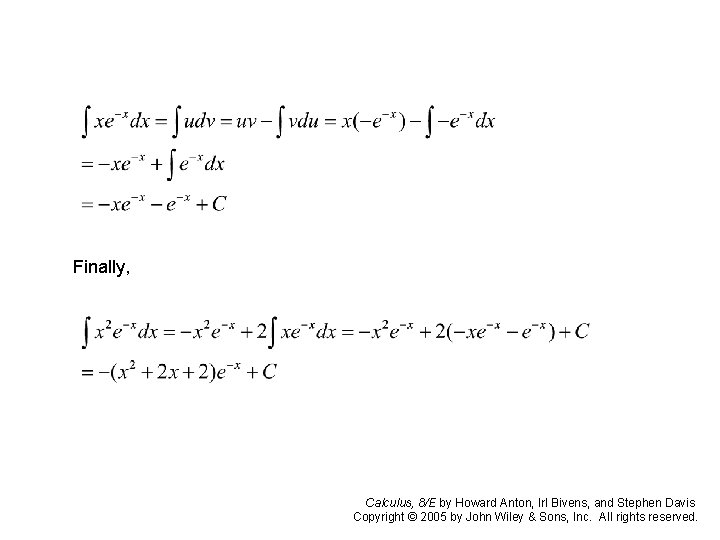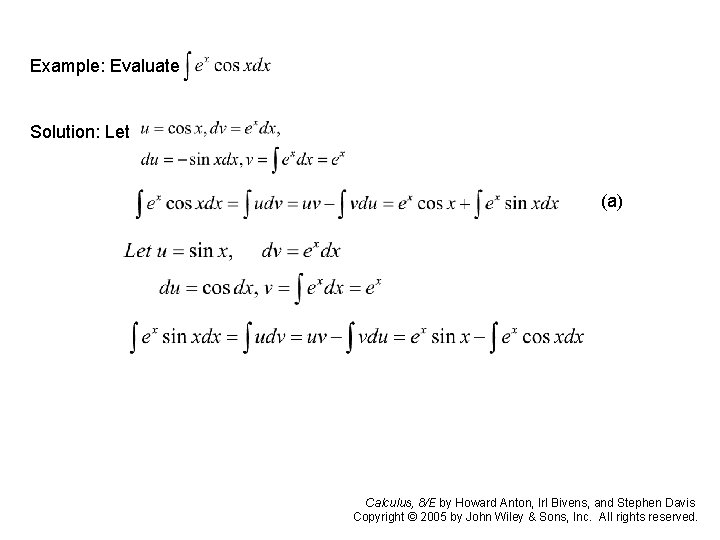Together with (a), we have Solve for the unknown integral, we have Calculus, 8/E by Howard Anton, Irl Bivens, and Stephen Davis Copyright © 2005 by John Wiley & Sons, Inc. All rights reserved.Integration by Parts for Definite Integrals For definite integrals, the formula corresponding to is Calculus, 8/E by Howard Anton, Irl Bivens, and Stephen Davis Copyright © 2005 by John Wiley & Sons, Inc. All rights reserved.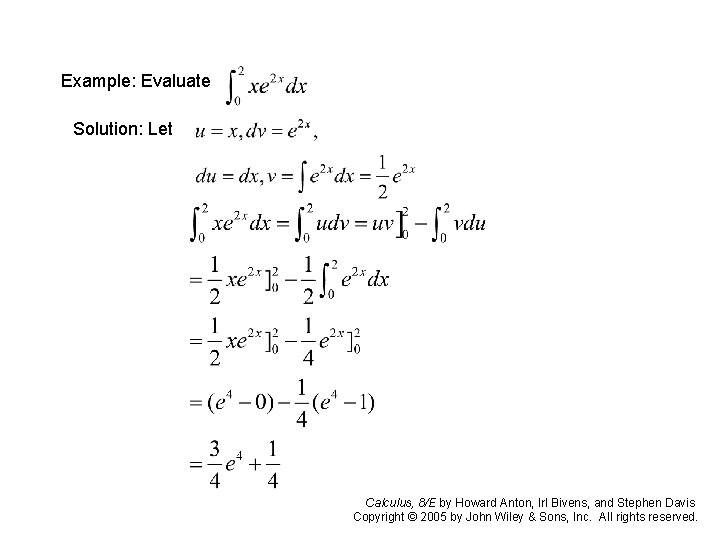8. 3 Trigonometric Integrals Integrating powers of sine and cosine By applying the integration by parts , we have two reduction formulas Calculus, 8/E by Howard Anton, Irl Bivens, and Stephen Davis Copyright © 2005 by John Wiley & Sons, Inc. All rights reserved.Integrating Products of Sines and Cosines Calculus, 8/E by Howard Anton, Irl Bivens, and Stephen Davis Copyright © 2005 by John Wiley & Sons, Inc. All rights reserved.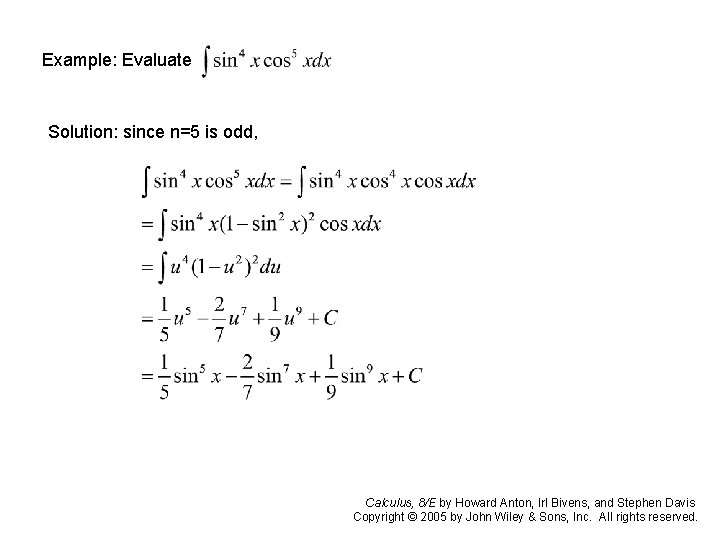Example: Evaluate Solution: since n=5 is odd, Calculus, 8/E by Howard Anton, Irl Bivens, and Stephen Davis Copyright © 2005 by John Wiley & Sons, Inc. All rights reserved.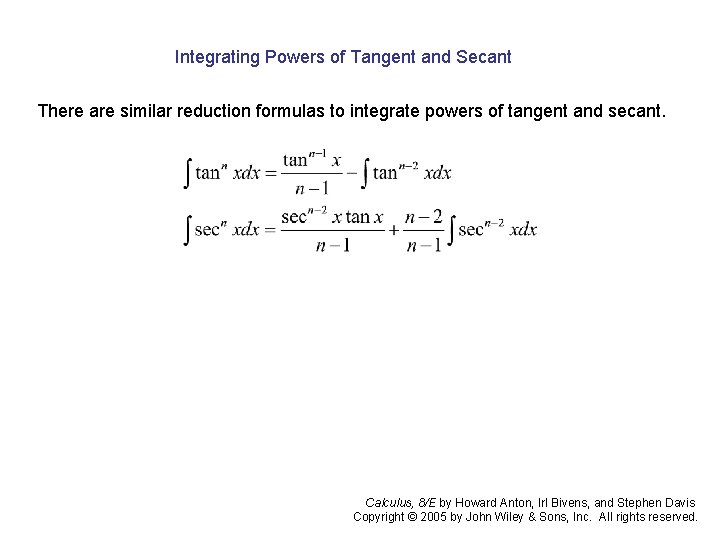Integrating Powers of Tangent and Secant There are similar reduction formulas to integrate powers of tangent and secant. Calculus, 8/E by Howard Anton, Irl Bivens, and Stephen Davis Copyright © 2005 by John Wiley & Sons, Inc. All rights reserved.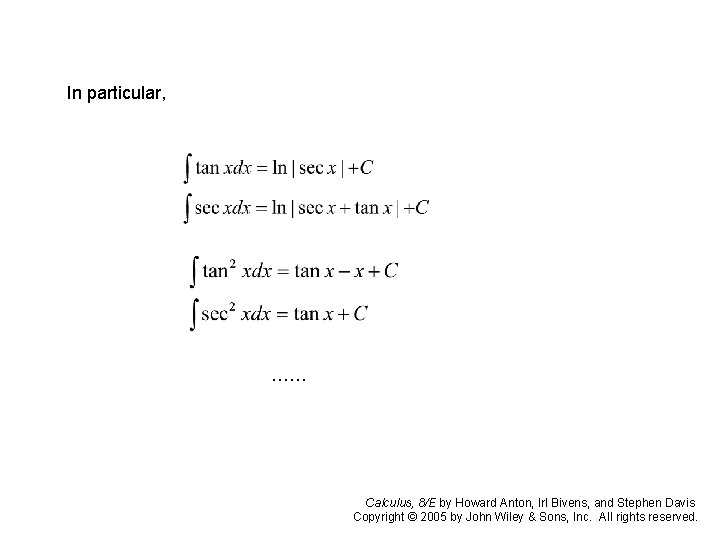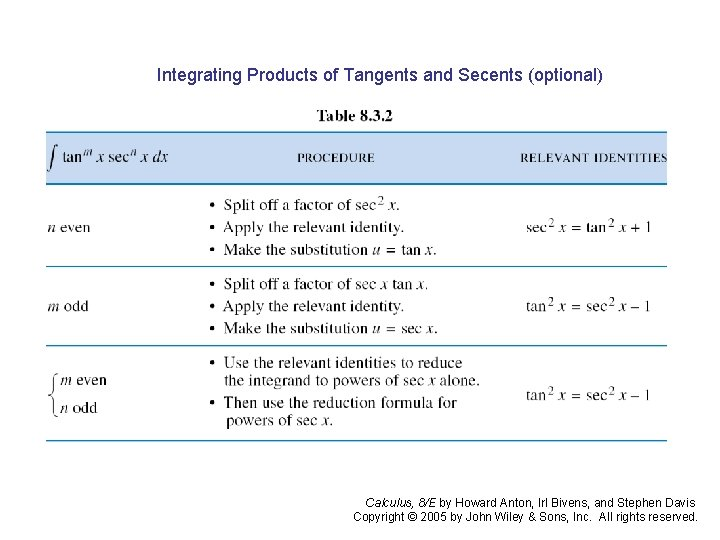Integrating Products of Tangents and Secents (optional) Calculus, 8/E by Howard Anton, Irl Bivens, and Stephen Davis Copyright © 2005 by John Wiley & Sons, Inc. All rights reserved.Section 8. 4 Trigonometric Substitutions We are concerned with integrals that contain expressions of the form In which a is a positive constant. The idea is to make a substitution for x that will eliminate the radical. For example, to eliminate the radical in , we can make the substitution x = a sinx, - /2 Which yields Calculus, 8/E by Howard Anton, Irl Bivens, and Stephen Davis Copyright © 2005 by John Wiley & Sons, Inc. All rights reserved.Example 1. Evaluate Solution. To eliminate the radical we make the substitution x= 2 sin , dx= 2 cos d This yields Calculus, 8/E by Howard Anton, Irl Bivens, and Stephen Davis Copyright © 2005 by John Wiley & Sons, Inc. All rights reserved.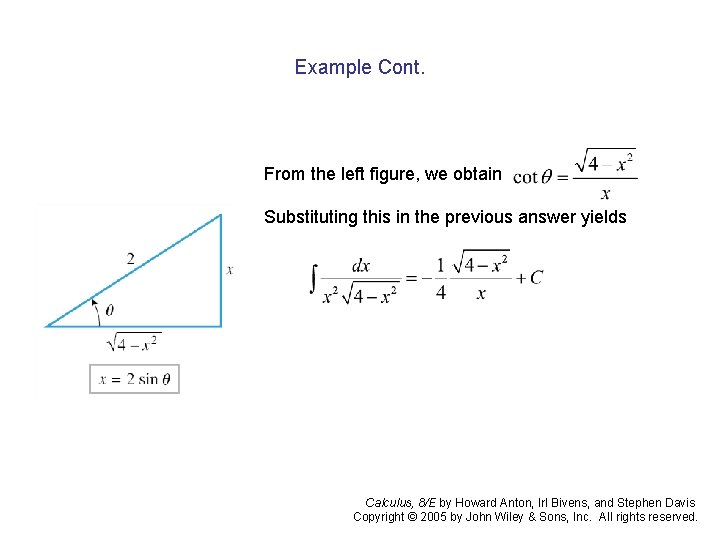Example Cont. From the left figure, we obtain Substituting this in the previous answer yields Calculus, 8/E by Howard Anton, Irl Bivens, and Stephen Davis Copyright © 2005 by John Wiley & Sons, Inc. All rights reserved.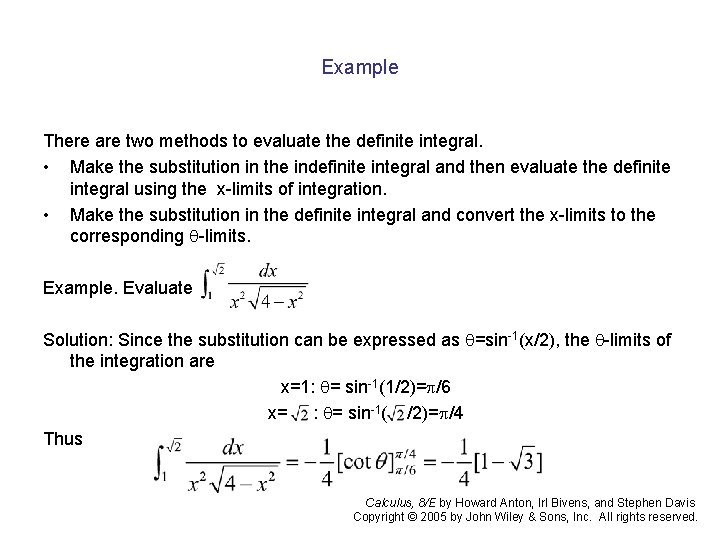Example There are two methods to evaluate the definite integral. • Make the substitution in the indefinite integral and then evaluate the definite integral using the x-limits of integration. • Make the substitution in the definite integral and convert the x-limits to the corresponding -limits. Example. Evaluate Solution: Since the substitution can be expressed as =sin-1(x/2), the -limits of the integration are x=1: = sin-1(1/2)= /6 x= : = sin-1( /2)= /4 Thus Calculus, 8/E by Howard Anton, Irl Bivens, and Stephen Davis Copyright © 2005 by John Wiley & Sons, Inc. All rights reserved.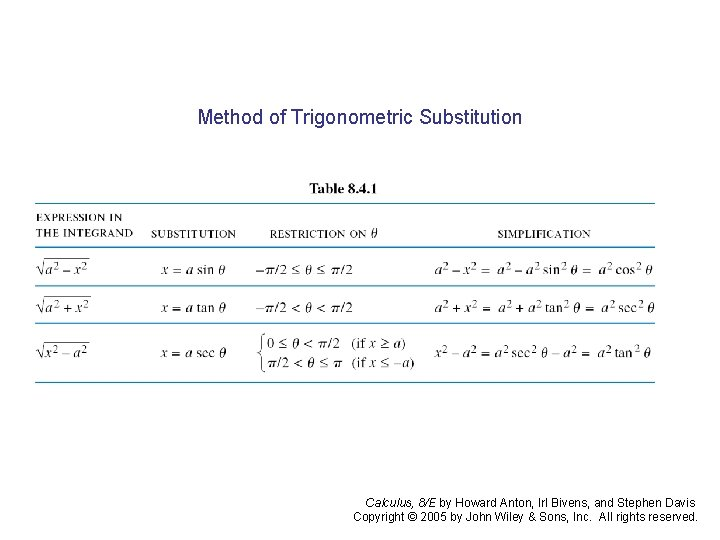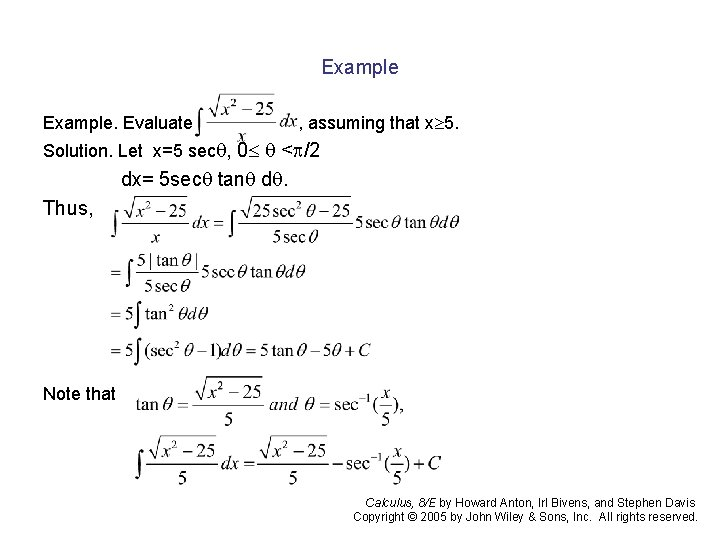Example. Evaluate , assuming that x 5. Solution. Let x=5 sec , 0 < /2 dx= 5 sec tan d. Thus, Note that Calculus, 8/E by Howard Anton, Irl Bivens, and Stephen Davis Copyright © 2005 by John Wiley & Sons, Inc. All rights reserved.8. 5 Integrating Rational Functions by Partial Fractionas Suppose that P(x) / Q(x) is a proper rational function, by which we mean that the Degreee of the numerator is less than the degree of the denominator. There is a Theorem in advanced algebra which states that every proper rational function can Be expressed as a sum Where F 1(x), F 2(x), …, Fn(x) are rational functions of the form In which the denominators are factors of Q(x). The sum is called the partial fraction Decomposition of P(x)/Q(x), and the terms are called partial fraction. Calculus, 8/E by Howard Anton, Irl Bivens, and Stephen Davis Copyright © 2005 by John Wiley & Sons, Inc. All rights reserved.Finding the Form of a Partial Fraction Decomposition The first step in finding the form of the partial fraction decomposition of a proper Rational function P(x)/Q(x) is to factor Q(x) completely into linear and irreducible Quadratic factors, and then collect all repeated factors so that Q(x) is expressed as A product of distinct factors of the form From these factors we can determine the form of the partial fraction decomposition Using two rules that we will now discuss. Calculus, 8/E by Howard Anton, Irl Bivens, and Stephen Davis Copyright © 2005 by John Wiley & Sons, Inc. All rights reserved.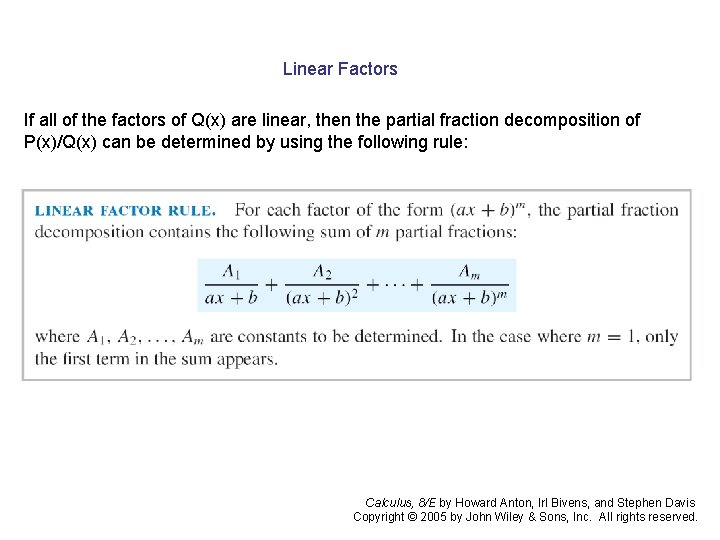Linear Factors If all of the factors of Q(x) are linear, then the partial fraction decomposition of P(x)/Q(x) can be determined by using the following rule: Calculus, 8/E by Howard Anton, Irl Bivens, and Stephen Davis Copyright © 2005 by John Wiley & Sons, Inc. All rights reserved.Example: Evaluate Solution: By the linear factor rule, the decomposition has the form Where A and B are constants to be determined. Multiplying this expression Through by (x-1)(x+2) yields 1=A(x+2)+B(x– 1) Let x=1, we have 1= 3 A which implies A = 1/3; and let x = -2, we have 1 = -3 B, Which means B = -1/3. Thus, Calculus, 8/E by Howard Anton, Irl Bivens, and Stephen Davis Copyright © 2005 by John Wiley & Sons, Inc. All rights reserved.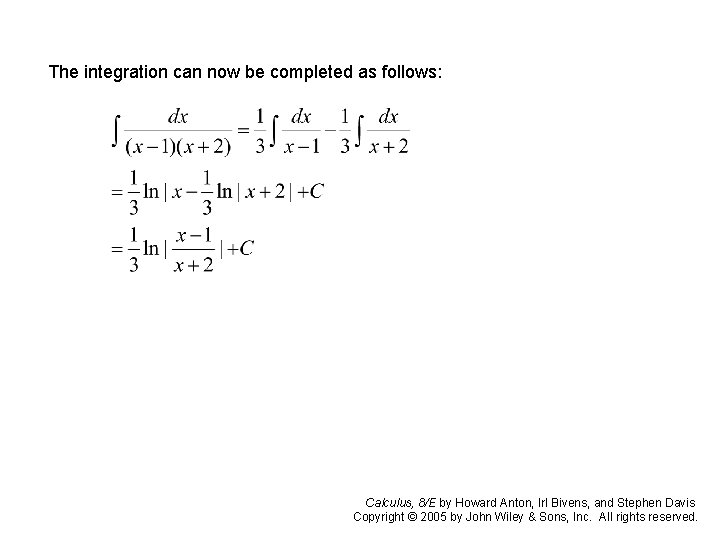The integration can now be completed as follows: Calculus, 8/E by Howard Anton, Irl Bivens, and Stephen Davis Copyright © 2005 by John Wiley & Sons, Inc. All rights reserved.Example: Evaluate Solution: By the linear factor rule, Multiplying by yields (1) (2) Setting x=0 in (1) yields B = -2, and setting x = 2 in (1) yields C = 2. Then By equating the coefficient of x 2 on the two sides to obtain A + C = 0 or A = -C = -2 Calculus, 8/E by Howard Anton, Irl Bivens, and Stephen Davis Copyright © 2005 by John Wiley & Sons, Inc. All rights reserved.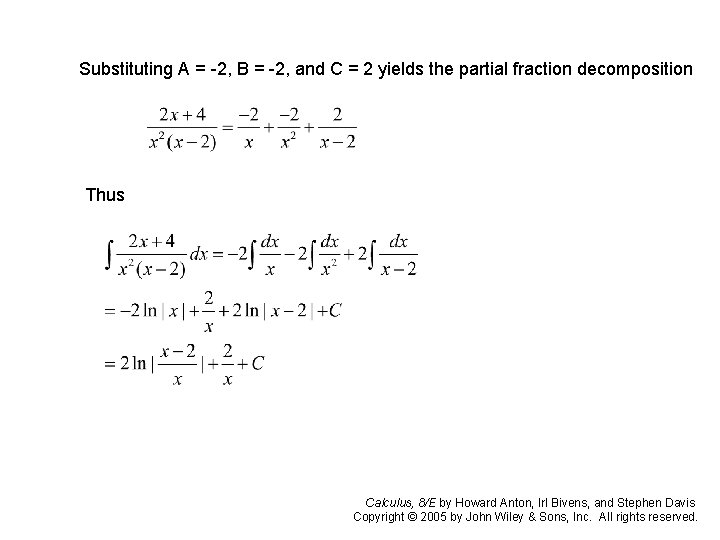Substituting A = -2, B = -2, and C = 2 yields the partial fraction decomposition Thus Calculus, 8/E by Howard Anton, Irl Bivens, and Stephen Davis Copyright © 2005 by John Wiley & Sons, Inc. All rights reserved.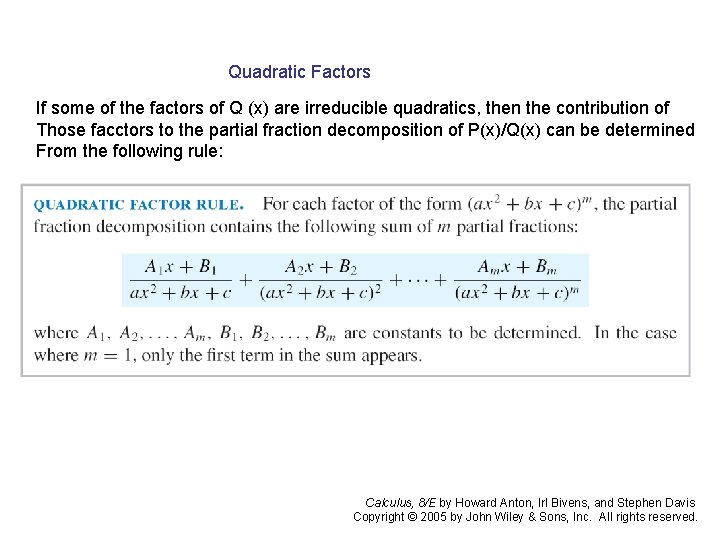Quadratic Factors If some of the factors of Q (x) are irreducible quadratics, then the contribution of Those facctors to the partial fraction decomposition of P(x)/Q(x) can be determined From the following rule: Calculus, 8/E by Howard Anton, Irl Bivens, and Stephen Davis Copyright © 2005 by John Wiley & Sons, Inc. All rights reserved.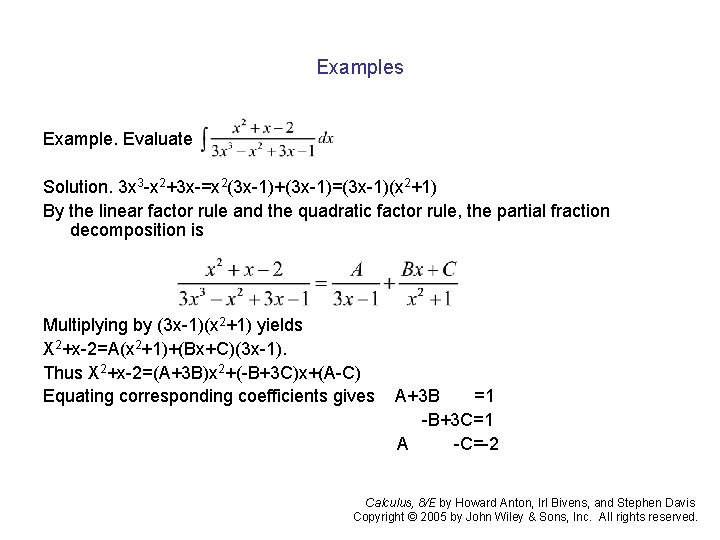Examples Example. Evaluate Solution. 3 x 3 -x 2+3 x-=x 2(3 x-1)+(3 x-1)=(3 x-1)(x 2+1) By the linear factor rule and the quadratic factor rule, the partial fraction decomposition is Multiplying by (3 x-1)(x 2+1) yields X 2+x-2=A(x 2+1)+(Bx+C)(3 x-1). Thus X 2+x-2=(A+3 B)x 2+(-B+3 C)x+(A-C) Equating corresponding coefficients gives A+3 B =1 -B+3 C=1 A -C=-2 Calculus, 8/E by Howard Anton, Irl Bivens, and Stephen Davis Copyright © 2005 by John Wiley & Sons, Inc. All rights reserved.Example Cont. By solving this, we get A=-7/5, B=4/5 and C=3/5. Thus Calculus, 8/E by Howard Anton, Irl Bivens, and Stephen Davis Copyright © 2005 by John Wiley & Sons, Inc. All rights reserved.